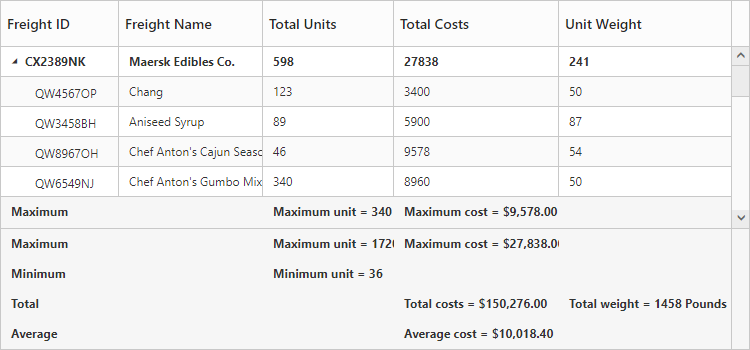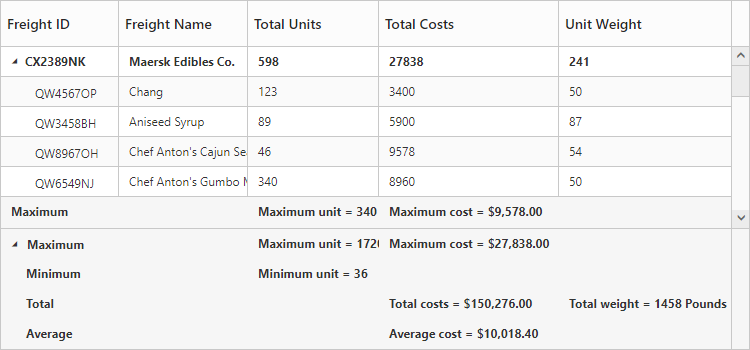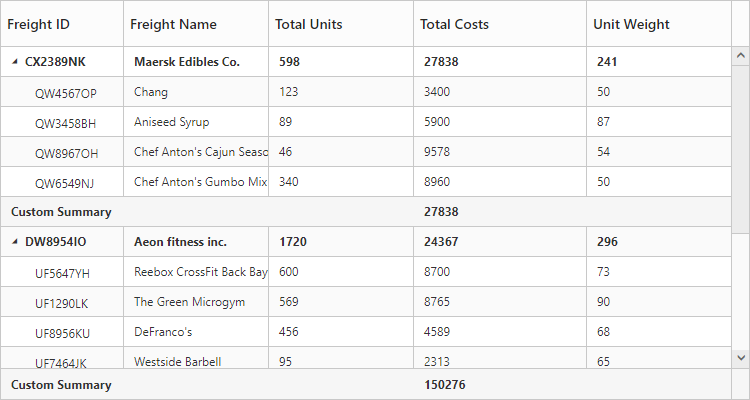# Summary Row

24 Sep 20185 minutes to read

Summary rows in TreeGrid is used to summarize every hierarchy with the set of predefined summary types using the column values.

• `e-tree-grid-summary-rows` - Using this property, user can define the summary rows in TreeGrid.
• `title` - Title for each summary row can be defined using this property.
• `e-tree-grid-summary-columns` - Using this property, it is possible to defined the summary for specific columns alone in a summary row.
• `show-summary-row` - This property is to make the summary row visible.
• `show-total-summary` - This property is to make the total summary row visible which is the overall summary row displayed for all the rows in the TreeGrid content.

## Defining summary columns

• `summary-type` - Using this property, user can define the type of summary to be displayed in a column.
• `datamember` - This property is used to map the field values which is used for summary calculations.
• `display-column` - This property is used to specify the column in which the summary to be displayed.
• `prefix` and `suffix` properties are used to define the text should be displayed along with the summary column value.
• `format` property is used for formatting the summary column value.

The below code snippet explains defining a summary row in TreeGrid,

• CSHTML
• ``````<ej-tree-grid id="TreeGridContainer" datasource="ViewBag.datasource" show-summary-row="true" show-total-summary="true" >
<e-tree-grid-summary-rows>
<e-tree-grid-summary-row title="Maximum">
<e-tree-grid-summary-columns>
<e-tree-grid-summary-column summary-type="Maximum" datamember="TotalUnits" display-column="TotalUnits" prefix="Maximum unit = "></e-tree-grid-summary-column>
<e-tree-grid-summary-column summary-type="Maximum" datamember="TotalCosts" display-column="TotalCosts" prefix="Maximum Cost = " format="{0:C}"></e-tree-grid-summary-column>
</e-tree-grid-summary-columns>
</e-tree-grid-summary-row>
<e-tree-grid-summary-row title="Total">
<e-tree-grid-summary-columns>
<e-tree-grid-summary-column summary-type="Sum" datamember="TotalCosts" display-column="TotalCosts" prefix="Total costs = " format="{0:C}"></e-tree-grid-summary-column>
<e-tree-grid-summary-column summary-type="Sum" datamember="UnitWeight" display-column="UnitWeight" prefix="Total weight = " suffix=" Pounds"></e-tree-grid-summary-column>
</e-tree-grid-summary-columns>
</e-tree-grid-summary-row>
</e-tree-grid-summary-rows>
</ej-tree-grid>``````

The below screenshot shows the output of above code example.## Customize height of total summary

Using `total-summary-height` property we can customize the height of the total summary container.
The below code example shows how to update the footer summary container height.

• CSHTML
• ``````<ej-tree-grid id="TreeGridContainer" datasource="ViewBag.datasource" show-total-summary="true" total-summary-height="120" >
//..
</ej-tree-grid>``````

The below screenshot shows the output of above code example.## Expand/collapse total summary row

We can expand/collapse the total summary rows in TreeGrid using following methods.

• Using Expander Icon
• Using Method

### Using Expander Icon

We can enable expander icon in total summary row by using `collapsible-total-summary` property. By default expander icon will be rendered in first row of 0th column in total summary rows.
Please find the below code example to enable collapsible total summary row in TreeGrid.

• CSHTML
• ``````<ej-tree-grid id="TreeGridContainer" datasource="ViewBag.datasource" show-total-summary="true" collapsible-total-summary="true" >
//..
</ej-tree-grid>``````NOTE

We can also customize the expander icon column in total summary row by using `_summaryColumnIndex` property and `load` event.

### Using Method

Total summary rows in TreeGrid can be expanded/collapsed by using `expandCollapseTotalSummary` method.
Please find the code example to collapse the total summary rows below.

• CSHTML
• ``````<button onclick="expandCollapse()">expandCollapse</button>
<ej-tree-grid id="TreeGridContainer" datasource="ViewBag.datasource" show-total-summary="true" collapsible-total-summary="true" >
//..
</ej-tree-grid>
function expandCollapse() {
var treeObj = \$("#TreeGridContainer").data("ejTreeGrid");
treeObj.expandCollapseTotalSummary(false);
}``````

## Custom Summary

Custom summary can be used to create summary values based on your required custom logic and calculations. To enable the custom summary, the `SummaryType` should be set to ‘Custom’ and the `CustomSummaryValue` property should be defined as function. After the custom calculation, the returned value will be displayed in the corresponding summary cell.

• HTML
• ``````<ej-tree-grid id="TreeGridContainer">
<e-tree-grid-summary-rows>
<e-tree-grid-summary-row>
<e-tree-grid-summary-columns>
<e-tree-grid-summary-column summary-type="Custom" custom-summary-value="sum" display-column="Duration">
</e-tree-grid-summary-column>
</e-tree-grid-summary-columns>
</e-tree-grid-summary-row>
</e-tree-grid-summary-rows>
</ej-tree-grid>``````
• JS
• ``````<script>
function sum(args, data) {
//ej.sum is aggregate to add data of total costs from datasource
return ej.sum(data, "TotalCosts");
}
</script>``````

The output of the tree grid with custom summary value is as follows.